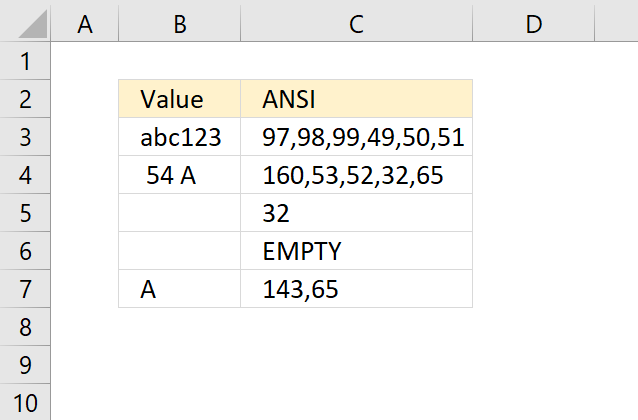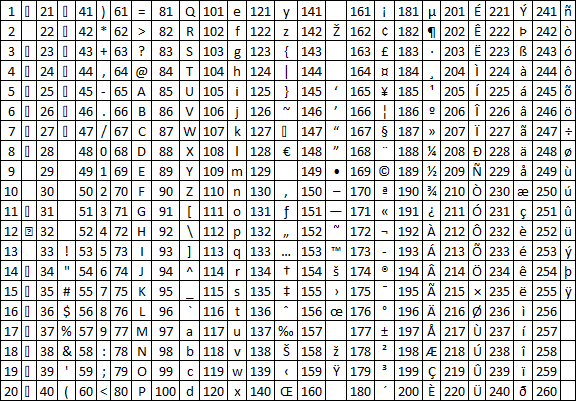Author: Oscar Cronquist Article last updated on April 19, 2022## 1. Identify all characters in a cell value

Sometimes when you sort values you get unexpected results, the cause is probably unwanted characters in a cell.

The same thing may happen if you try to trim blanks in a cell value and it fails to remove the character that looks like the space character.

The TRIM function removes only ANSI character 32, however, the HTML blank character number 160 is not removed by TRIM.

The following array formula demonstrated in cell C3 in the picture above will convert each character in a cell value to its corresponding ANSI number (PC).

=IFERROR(TEXTJOIN(",", TRUE,CODE(MID(B3, ROW(\$A\$1:INDEX(\$A\$1:\$A\$1000, LEN(B3))), 1))), "EMPTY")

This will make it easier for you to see all the characters that cause problems. Cell B3 contains only letters and numbers, nothing weird.

Cell B5 contains a single space character, code 32. However, cell B4 contains an HTML character that looks like a space character. Cell B7 also has a weird space character, code 143.

As you can see the formula above assists you in finding weird characters.

### Explaining formula in cell C3

LEN(B3)

#### Step 2 - Create cell reference to a cell

INDEX(\$A\$1:\$A\$1000, LEN(B3))

#### Step 3 - Create a cell reference to a cell range

The INDEX function and the LEN function allows you to create a cell reference with as many rows as there are characters in cell C3.

\$A\$1:INDEX(\$A\$1:\$A\$1000, LEN(B3))

becomes

\$A\$1:INDEX(\$A\$1:\$A\$1000, 6)

and returns

\$A\$1:\$A\$6.

#### Step 4 - Create a sequential list of numbers from 1 to n

The ROW function then creates an array from 1 to the number of characters in cell C3.

ROW(\$A\$1:INDEX(\$A\$1:\$A\$1000, LEN(B3)))

becomes

ROW(\$A\$1:\$A\$6)

and returns

{1;2;3;4;5;6}

#### Step 5 - Split characters into an array

Now it is time for the MID function to split each character in cell C3 to an array.

MID(B3, ROW(\$A\$1:INDEX(\$A\$1:\$A\$1000, LEN(B3))), 1)

becomes

MID("abc123", {1;2;3;4;5;6}, 1)

and returns

{"a";"b";"c";"1";"2";"3"}

#### Step 6 - Convert characters into ANSI numbers

The CODE function allows you to convert a character to ANSI code (PC). We are using an array so the function will convert all characters at the same time.

CODE(MID(B3, ROW(\$A\$1:INDEX(\$A\$1:\$A\$1000, LEN(B3))), 1)))

becomes

CODE({"a";"b";"c";"1";"2";"3"})

and returns

{97;98;99;49;50;51}.

#### Step 7 - Join values in the array

Lastly, the TEXTJOIN function concatenates all values in the array with the delimiting character , (comma).

TEXTJOIN(",", TRUE,CODE(MID(B3, ROW(\$A\$1:INDEX(\$A\$1:\$A\$1000, LEN(B3))), 1)))

becomes

TEXTJOIN(",", TRUE,{97;98;99;49;50;51})

and returns

97,98,99,49,50,51 in cell C3.

#### Step 8 - Catch errors

IFERROR(TEXTJOIN(",", TRUE,CODE(MID(B3, ROW(\$A\$1:INDEX(\$A\$1:\$A\$1000, LEN(B3))), 1))), "EMPTY")

If the cell value is empty the formula returns #VALUE, the IFERROR function then displays "EMPTY".

### ANSI tableThe picture above shows characters for number 1 to 255 (ANSI).

## 2. Identify all characters in a cell value - Excel 365Excel 365 dynamic array formula in cell C3:

=IFERROR(TEXTJOIN(",", TRUE,CODE(MID(B3, SEQUENCE(LEN(B3)), 1))), "EMPTY")

### 2.1 Explaining formula

LEN(B3)

returns 6.

#### Step 2 - Create a sequential list of numbers from 1 to n

SEQUENCE(LEN(B3))

returns

{1;2;3;4;5;6}

#### Step 3 - Split characters into an array

Now it is time for the MID function to split each character in cell C3 to an array.

MID(B3, SEQUENCE(LEN(B3)), 1)

becomes

MID("abc123", {1;2;3;4;5;6}, 1)

and returns

{"a";"b";"c";"1";"2";"3"}

#### Step 4 - Convert characters into ANSI numbers

The CODE function allows you to convert a character to ANSI code (PC). We are using an array so the function will convert all characters at the same time.

CODE(MID(B3, SEQUENCE(LEN(B3)), 1))

becomes

CODE({"a";"b";"c";"1";"2";"3"})

and returns

{97;98;99;49;50;51}.

#### Step 5 - Join values in the array

Lastly, the TEXTJOIN function concatenates all values in the array with the delimiting character , (comma).

TEXTJOIN(",", TRUE,CODE(MID(B3, SEQUENCE(LEN(B3)), 1)))

becomes

TEXTJOIN(",", TRUE,{97;98;99;49;50;51})

and returns

97,98,99,49,50,51 in cell C3.

#### Step 6 - Catch errors

IFERROR(TEXTJOIN(",", TRUE,CODE(MID(B3, SEQUENCE(LEN(B3)), 1))), "EMPTY")

If the cell value is empty the formula returns #VALUE, the IFERROR function then displays "EMPTY".

### Get Excel *.xlsx file

Identify characters in a cell value.xlsx